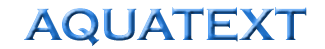Channel Flow Calculation - Mannings equation

 v = 1/n x R2/3 x S1/2 Where : n = coefficient of roughness (typically 0.3) v = Water velocity down the channel (m / sec) = flow (m3sec-1) / cross sectional area (m2) R = Hydraulic radius (m) = cross sectional area (m2) / wetted perimeter (m) S = Gradient of channel (m / 100m)